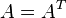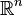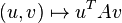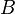# Symmetric positive-definite matrix

Jump to: navigation, search
This article defines a property that can be evaluated for a square matrix with entries over the field of real numbers. In other words, given a square matrix (a matrix with an equal number of rows and columns) with entries over the field of real numbers, the matrix either satisfies or does not satisfy the property.
View other properties of square matrices with entries over the field of real numbers | View other properties of square matrices

## Definition

Supposeis a positive integer andis asquare matrix. We say thatis a symmetric positive-definite matrix if the following equivalent conditions hold:

1. 'Symmetric and positive-definite:(i.e.,is a symmetric matrix: it equals its matrix transpose) andis a positive-definite matrix: for everycolumn vector, we have that, and equality holds if and only ifis the zero vector (in other words,for all nonzerocolumn vectors).
2. The bilinear form ondefined by(where the input vectors are written as column vectors) is a symmetric positive-definite bilinear form.
3. There is ainvertible matrixsuch that.
4.is a symmetric matrix and a P-matrix.

## Relation with other properties

### Weaker properties

Property Meaning Proof of implication Proof of strictness (reverse implication failure) Intermediate notions
invertible matrix |
positive-definite matrix |
P-matrix |